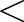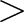Data Science Desktop Survival Guide by Graham WilliamsDesktop Survival Project Home Preface Data Science Introducing R R Constructs R Tasks R Strings R Read, Write, and Create Data Template Data Exploration Data Wrangling Data Visualisation Statistics ML Template ML Scenarios ML Activities ML Applications ML Algorithms Cluster Analysis Decision Trees Computer Vision Graph Data Privacy Literate Data Science Coding with Style Resources Bibliography Index

## Assignment

20200105

resume
Avoid using base::= for assignment. It was introduced in S-Plus in the late 1990s as a convenience but is ambiguous (named arguments in functions, mathematical concept of equality). The traditional backward assignment operator base::- implies a flow of data and for readability is explicit about the intention.

Preferred

 a <- 42 b <- mean(x) Discouraged
 a = 42 b = mean(x) The forward assignment base::-should generally be avoided. A single use case justifies it in pipelines where logically we do an assignment at the end of a long sequence of operations. As a side effect operator it is vitally important to highlight the assigned variable whenever possible and so out-denting the variable after the forward assignment to highlight it is recommended. Preferred
 ds[vars] %>%   sapply(function(x) all(x == x[1L])) %>%   which() %>%   names() %T>%   print() -> constants Traditional
 constants <-   ds[vars] %>%   sapply(function(x) all(x == x[1L])) %>%   which() %>%   names() %T>%   print() Discouraged
 ds[vars] %>%   sapply(function(x) all(x == x[1L])) %>%   which() %>%   names() %T>%   print() ->   constants